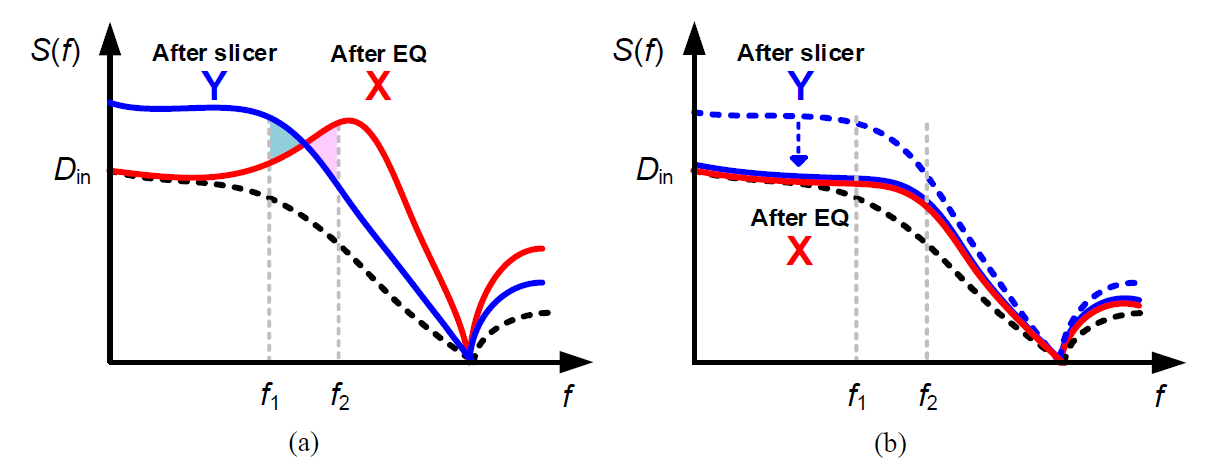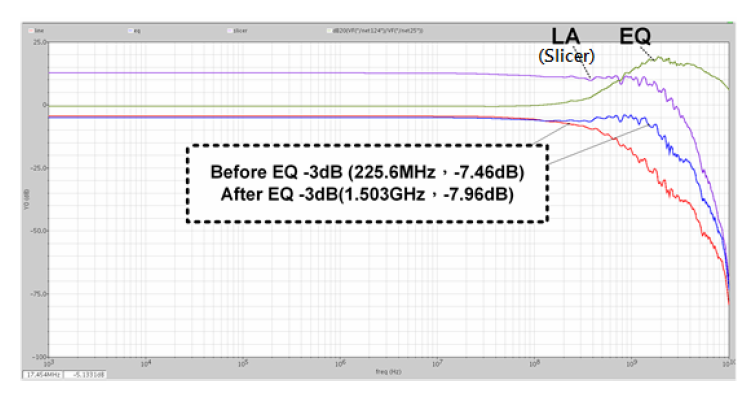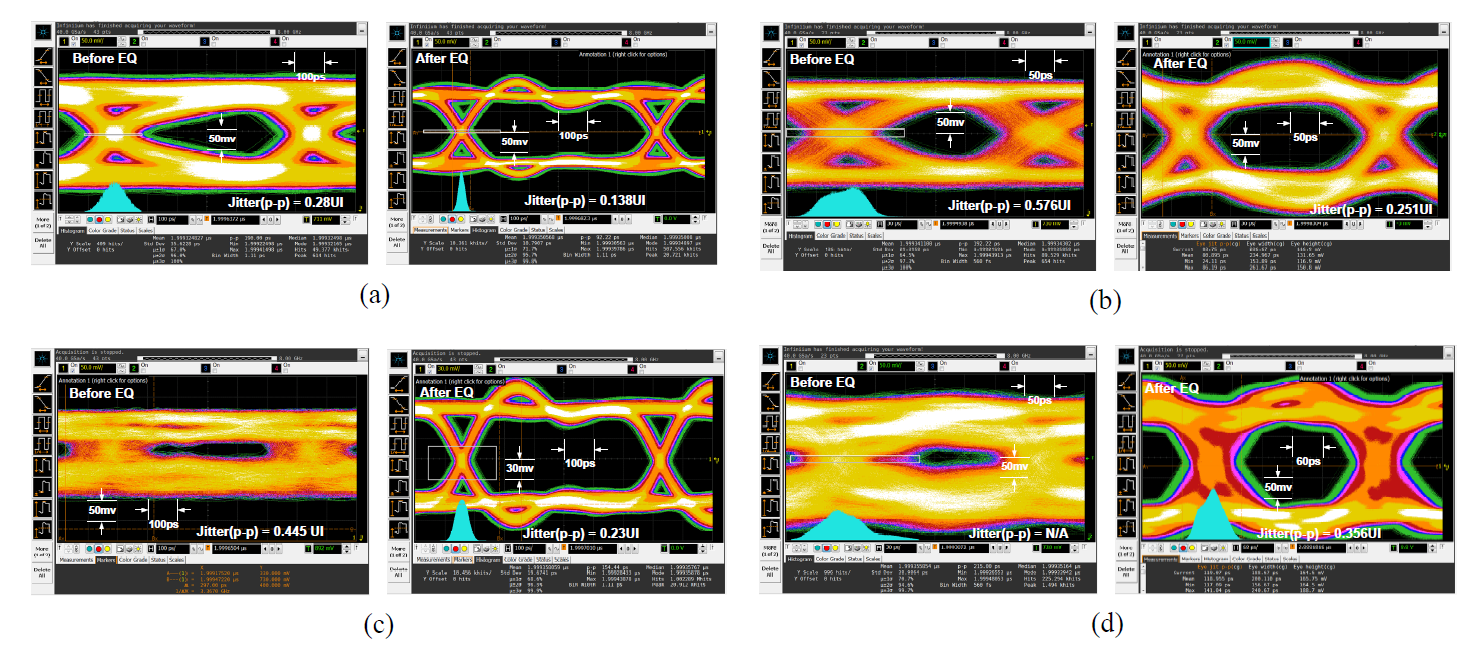Mobile QR Code1. (Department of Electrical Engineering, National Chung Hsing University, Taichung City, Taiwan (ROC))
2. (ILI Technology Corp., Hsinchu Country, Taiwan (ROC))

Intra-panel interface, TFT-LCD, UHD, adaptive equalizer, swing controller

## I. INTRODUCTION

The video streaming applications develop rapidly between mobile devices and TVs, especially for the next generation TFT-LCD devices with Ultra HD (UHD) 4K2K (3840 ´ 2160) resolution. As a result, high-speed interface becomes a critical design. For example, Fig. 1 shows the UHD display system with intra-panel interface, where the length and width are two times of the FHD resolution. As the TFT-LCD panel becomes larger, the transmission distance between timing controller (TCON) and column drivers (CD) becomes longer. Thus, the dielectric loss and skin effect results in much more serious in high-speed transmission, which will cause inter-symbol interference (ISI) issue. In order to solve above problems, an adaptive equalizer is indeed for the display system.

Several adaptive methods for the continuous time equalizer (CTLE) have been proposed. The conventional architecture with two high-pass filters (HPF) will cause an unbalancing-swing problem between input and output of the slicer. Although the enhanced low-frequency gain method with two low-pass filters (LPF) can adjust the low-frequency gain of the equalizer. The highfrequency gain tuning range will be limited because the trade-off between high frequency and low frequency compensations in the equalizer filter. In order to solve the unbalancing swing problem, the spectrum balancing technique has been proposed[3,4], which removes the slicer and sensing the energy of equalizer’s output directly with HPF and LPF for lower power applications. However, this method works only if the data is random and fixed data rate. For the intra-panel interface of the display system, we must find a more robust design for the equalizer.

In order to solve the problems above, an adaptive equalizer with swing control technique was proposed[5-7]. In this work, we adopt a dual control technique using a swing-matching balancer for the slicer and an adaptive controller to tune the gain and bandwidth for the frontend equalizer. By generating swings that match the equalizer output swings, it won’t have the unbalancing swing problem. Furthermore, in order to overcome the various transmission distance between TCON and CD of UHD 4K2K large display panel. We add a fine tune loop to adjust the low-frequency gain by 3-bit resistor-string DAC, which can not only ensure the equalizer have an enough wide bandwidth for various loss but also overcome the variations of process, voltage, and temperature (PVT).

Section II presents the architecture and operation of the proposed adaptive equalizer. Section III describes the circuit in detail. Section IV and V show the measurement results and conclusion, respectively.

## II. ARCHITECTURE

Fig. 2 shows the block diagram of the proposed adaptive equalizer, where the equalizer’s filters followed by a slicer. The high-frequency compensation is sensing signal X and Y by two pairs of band-pass filters (BPF), which measuring the energy in a narrow range of frequencies to promote the accuracy. Then the rectifiers extract the high-frequency power. After that, the difference between these two signals is amplified by the error amplifier to generate a feedback signal (Vctrl_High) for the equalizer filter. In order to eliminate the unbalancing swing problem that cause by the signal level and circuit differences between signal X and Y, we generate a feedback signal (Vctrl_Swing) to control slicer tail current. Besides, another signal (Vctrl_Adj) is generated to overcome the various loss cause by different lengths of FR4 board (from 23 cm to 123 cm) and PVT variations by a 3-bit resistor string DAC.

In order to prove the system stability, we assume there is only one compensated loop to adjust the equalizer by BPF. At the stable condition, we can find out the spectrum at X and Y as blow:

##### (1)
$S_{X}(f)=K_{X} T_{b}\left(\frac{\sin \left(\pi f T_{b}\right)}{\pi f T_{b}}\right)^{2} \frac{1}{f^{2} / f_{p 1}^{2}+1}$

##### (2)
$S_{Y}(f)=K_{Y} T_{b}\left(\frac{\sin \left(\pi f T_{b}\right)}{\pi f T_{b}}\right)^{2} \frac{1}{f^{2} / f_{p 2}^{2}+1}$

where KX and Ky are the scaling factors of the signals X and Y, and Tb is the bit period. As shown in Fig. 3, it measure the energy in narrow range of $f_{1}$ and $f_{2}$ by BPF, and we can express as:

Fig. 3. Spectrum of the equalizer (a) without; (b) with the swing controller.##### (3)
$\int_{f 1}^{f 2} S_{X}(f) d f=\int_{f 1}^{f 2} S_{Y}(f) d f$

In this condition, the output of X and Y carry similar spectra, which will cause poor adaptation, even if the error in the adaptive loop is approach zero. Because the spectrum of X between $f_{1}$ and $f_{2}$ is determined by the voltage swing of X. Besides, the voltage swings between X and Y must be different, which cause by characteristics of different length of transmission line and TX driver circuit.

The unbalancing problem can be alleviated by adjusting the equalizer low-pass frequency gain directly, but it will limit the high-frequency gain relatively. Increasing a swing control loop as the architecture in Fig. 2. We assume the low-pass characteristics of the signal X and Y is also captured in (1) and (2), which have the - 3-dB frequencies of $f_{p1}$ and $f_{p2}$ , respectively. Thus, for the swing control loop it can be expressed as:

##### (4)
$\int_{0}^{f_{0}} S_{X}(f) d f=\int_{0}^{f_{0}} S_{Y}(f) d f$

where $f_{0}$ is the -3-dB frequency of the “assumable” LPF. When the stable state satisfy the following conditions, $K_{X} \approx K_{Y}$ , and $f_{p 1} \approx f_{p 2}$ . We can modified (4) as blow:

##### (5)
$\int_{0}^{\infty} S_{X}(f) d f=\int_{0}^{\infty} S_{Y}(f) d f$

As shown in Fig. 3(b), the result shows the signal X (after equalizer) smoothly than in Fig. 3(a). In this way, we can achieve the same goal without LPF for saving chip area and power consumption. Furthermore, it won’t limit the tuning range of high-frequency gain, which can improve the accuracy of the adaptation.

Unlike the conventional spectrum-balancing technique only works for random and fixed data rate. In the proposed architecture, the control for the high-frequency gain won’t interfere by unbalancing swing problem, but also suits for any coding scheme at the transmitter including the clock-embedded coding technique for intrapanel interface. As the advantages above, the architecture is very suitable for the next generation UHD 4K2K display panel, which have a various transmission distance.

## III. CIRCUIT DESCRIPTION

### 1. Equalizer Filter

The equalizer filter is composed of three cascaded filter cells to obtain enough high-frequency gain and bandwidth. Fig. 4 shows the schematic of single-stage equalizer filter, where the controlled voltage of Cvar, Vctrl_High, is used to provide boost tuning range. Unlink the conventional equalizer, in Fig. 4 the controlled voltage of M3 , Vctrl_Adj, is used to adjust low-frequency gain of equalizer filter. In  and , the feedback architecture was adopted to effectively compensate power loss of incoming data. The received input data goes through an equalizing filter and the equalizing filter roughly compensates for power loss at high frequencies with the initial filter gain. In this work, we improve the equalizer in  and  by adding a 3-bit DAC in Fig. 4 for course tuning to enhance the tuned bandwidth for various loss and PVT variations. As a result, the proposed circuit can mitigate the trade-off between high-frequency and low-frequency gains of the equalizer filter. Fig. 5. shows the frequency response of the 3-stage equalizer filter by controlling the voltage (Vctrl_High) when the 3-bit code is 1-0-0. The boost tuning range can achieve from 22.58 to 9.4 dB at 1.5 GHz when the controlled voltage is from 0 to 1.8 V.

### 2. Control Loop with Rectifier and Error Amplifier

The detector plays a critical role at adaptive adaptation loop. The conventional rectifier will have errors cause by the common-mode mismatch and the different input swing. In order to solve these problems, two rectifiers share the same current source ( Iss ). Besides, we use fixed resistor ( Rs ) at source-coupled node, as shown in Fig. 6, which has the advantage that the mismatch between the two paths is minimized even if the common mode is different. After rectifying the differential outputs of the two band-pass filters, the signals are then averaged by low-pass filter, which prevents slewing of the error amplifier and hence helps maintain a relatively high loop gain, while averaging the rectified signal. Moreover, the corresponding difference between the two paths is amplified by a two-stage error amplifier, which uses the negative resistor of Mn2-Mn3 source-coupled pair. It can reach to the better loop gain, approximately equal to 51 dB.

### 3. Slicer

The slicer is the limiting amplifier (LA) composed of cascaded amplifiers, illustrated in Fig. 7. The slicer can sharpen rising and falling edges of data from the equalizing filter. The slicer generally requires high gain and wide bandwidth characteristics. The natural bandwidth of the designed amplifying stage decreases when stages are cascaded. As a result, we choose a three-stage differential amplifier as the slicer according to above considerations. The output swing can be adjusted by the tail current Itail, which is controlled by the feedback voltage Vctrl_Swing.

## IV. SIMULATED AND MEASURED RESULTS

The adaptive equalizer was fabricated in 0.18-μm CMOS technology. The die photograph of the chip is shown in Fig. 8. The total chip area is 0.47 mm2, and the core area is 0.12 mm2. The S21 parameters of various lengths of FR4 board are shown in Fig. 9, from - 6 dB to - 24 dB at 1.5 GHz. Fig. 10 shows the simulated frequency response of the whole system, the - 3 dB bandwidth from 225.6 MHz to 1.5 GHz after equalization. To evaluate the circuit performance, the prior work using the die on package can reach to 1.5 Gb/s. Alternatively, we adopted the die on PCB to reduce the parasitic effects of interface and the data rate of the proposed equalizer can achieve to 3 Gb/s. The test channel was a 1.23-m trace on the PCB with 24-dB loss at 3 Gb/s. Fig. 11(a)-(d) show the measured eye diagrams before and after equalization at 1.5-, and 3-Gb/s 27 -1 PRBS pattern for 0.56-m and 1.23-m channel lengths, respectively. The measured equalized signals have only 0.356-UI peak-to-peak jitter at 3-Gb/s. The equalizer chip consumes 27-mW with 1.8-V power supply at 3-Gb/s, excluding the output buffer. Table 1 shows the performance summary and the comparison with the prior works[2-4,6,8-11].

Fig. 10. Simulated frequency response of adaptive equalizer.Fig. 11. Eye diagrams of (a) 1.5-Gb/s, (b) 3-Gb/s 27 -1 PRBS pattern of a 0.56-m channel length, and eye diagrams of (c) 1.5-Gb/s, (d) 3-Gb/s 27 -1 PRBS pattern of a 1.23-m channel length.Table 1. Performance summary and comparison

 Ref. This work 2004  2009  2014  2012  2012  2017 * 2018  2018  Simulation Measurement Technology ($\mu$m) 0.18 CMOS 0.18 CMOS 0.5 SiGe 0.13 CMOS 0.18 CMOS 0.13 CMOS 0.18 CMOS 0.09 CMOS 0.13 CMOS Supply voltage (V) 1.8 1.8 3.3 3.3 1.8 NA 1.8 1.0 1.8 Channel loss (dB) -24@1.5GHz ; -13@0.75GHz -16 -17 -20 -17 -16 -20 -13.65 -7 Power consumption (mW) 27 25.75 80 108 34 22.3 35 36.8 4.35 81.7 Data rate (Gb/s) 3/1.5 3.5 2.25 5 2.7 5.4 5.4 5 5 Jitter (UI) 0.256/0.14 0.356/0.23 0.39 0.1 0.36 0.48 0.81 0.68 0.36 0.23 Area (mm2) 0.12 0.35 0.25 0.24 0.11 0.18 0.265 0.67 0.36 Efficiency (pJ/bit) 9 8.6 22.9 48 6.8 8.26 6.48 6.81 0.87 10.2

* including the equalizer and clock and data recovery (CDR)

## V. CONCLUSIONS

An equalizer with adaptive swing controller for the next generation 4K2K resolution TVs has been presented. It can improve the adaptation accuracy without degrades the boost tuning and input swing ranges. Furthermore, adding a 3-bit resistor string DAC for the equalizer filter is not only able to compensate for wide range of various lengths, but also immune from the PVT variations. The chip was fabricated in 0.18-μm CMOS process. The proposed adaptive equalizer shows the output peak-topeak jitter is 0.356-UI at 3-Gb/s of 24-dB channel loss (1.23-m PCB length).

### ACKNOWLEDGMENTS

The authors would like to thank the Ministry of Science and Technology (MSOT) and the ILI Technology Corp., Taiwan (ROC), for the financial support, and the National Chip Implementation Center (CIC), Taiwan (ROC), for the infrastructure support.

### REFERENCES

1
Baker A. J., Feb. 1996, An adaptive cable equalizer for serial digital video rates to 400Mb/s, IEEE Int. Solid-State Circuits Conf. Dig. Tech. Papers, pp. 174-1752
Choi J. S., Hwang M. S., Jeong D. K., Mar. 2004, A 0.18-μm CMOS 3.5-Gb/s continuous-time adaptive cable equalizer using enhanced low-frequency gain control method, IEEE J. Solid-State Circuits, Vol. 39, No. 3, pp. 419-4253
Liu H., Mohammed I., Fan Y., Morgan M., Liu J., Jul. 2009, An HDMI cable equalizer with self-generated energy ratio adaptation scheme, IEEE Trans. Circuits Syst. II, Exp. Briefs, Vol. 56, No. 7, pp. 595-5994
Liu H., et al , Apr. 2014, A 5-Gb/s serial-link redriver with adaptive equalizer and transmitter swing enhancement, IEEE Trans. Circuits Syst. I, Reg., Vol. 61, No. 4, pp. 1001-10115
Gondi S., Razavi B., Sep. 2007, Equalization and clock and data recovery techniques for 10-Gb/s CMOS serial-link receivers, IEEE J. Solid-State Circuits, Vol. 42, No. 9, pp. 1999-20116
Lee W. Y., Kim L. S., May 2012, An adaptive equalizer with the capacitance multiplication for DisplayPort main link in 0.18-μm CMOS, IEEE Trans. Very Large Scale Integr. Syst., Vol. 20, No. 5, pp. 964-9687
Lin Y. C., Yang C. Y., Chang J., Nov. 2017, A 1.5-Gb/s equalizer with adaptive swing controller for TFTLCD driver, International SoC Design Conference (ISOCC 2017), pp. 250-2518
Kim W. S., Seong C., Choi W. Y., Sep. 2012, A 5.4-Gbit/s adaptive continuous-time linear equalizer using asynchronous undersampling histograms, IEEE Trans. Circuits Syst. II, Express Briefs, Vol. 59, No. 9, pp. 553-5579
Hwang S., Song J., Lee Y., Kim C., Oct. 2017, A 1.62–5.4-Gb/s receiver for displayport version 1.2a with adaptive equalization and referenceless frequency acquisition techniques, IEEE Trans. Circuits Syst. I, Reg. Papers, Vol. 64, No. 10, pp. 2691-270210
Tu Y. H., Cheng K. H., Lee M. J., Liu J. C., Jul. 2018, A power-saving adaptive equalizer with a digitalcontrolled self-slope detection, IEEE Trans. Circuits Syst. I, Reg. Papers, Vol. 65, No. 7, pp. 2097-210811
Wu H., Wang J., Liu H., April 2018, A 5 Gb/s CMOS adaptive equalizer for serial link, J. Semiconductors, Vol. 39, No. 4, pp. 045003-1-045003-6## Author

was born in Tainan, Taiwan, in 1991.

She received the B.S. degree from National Yunlin University of Science and Technology, Taiwan, in 2013, and the M.S. degree from the National Chung Hsing University, Taiwan, in 2016.

She is currently working toward the Ph.D. degree in Graduate Institute of Electrical Engineering, National Chung Hsing University, Taiwan.

Her research interests include phase-locked loop and clock and data recovery circuits.

was born in Kaohsiung, Taiwan, in 1993.

He received the B.S. degree in electronic engineering from Tunghai University, Taichung, Taiwan, in 2015, and the M.S. degree in electronic engineering from the National Chung Hsing University, Taichung, Taiwan, in 2018.

His research interests include phase-locked loop and high-speed interfaces.

was born in Taoyuan, Taiwan, in 1992.

He received the B.S. degree in electronic engineering from National Yunlin University of Science and Technology, Yunlin, Taiwan, in 2015, and the M.S. degree in electronic engineering from the National Chung Hsing University, Taichung, Taiwan, in 2018.

His research interests include phase-locked loop and high-speed interfaces.

was born in Taiwan, R.O.C., in 1974.

He received the B.S., M.S. and Ph.D. degrees from National Chung Cheng University, Taiwan, in 1996, 1998, and 2002 respectively. During 2002-2007, he was with Infineon Taiwan, engaged in the mixed-signal designs, which including E-PHY, USB, Wireless LAN, and DDR.

He joined ILITEK in 2007, working on display driver related circuits, where he is currently a Deputy Director.

His research interests include high-speed and low-power digital integrated circuits, analog integrated circuits, high speed serial interface and display related circuits design.

was born in Taiwan, in 1993.

He received the B.S. degree in electrical engineering from National Chung Hsing University, Taiwan.

He is currently studying for M.S. degree in National Chung Hsing University, Taiwan.

He currently researches on high-speed interface transmission system.

was born in Taipei, R.O.C., in 1994.

He received the B.S. degree in electrical and computer engineering from TamKang University, Taipei, R.O.C., in 2017.

He is currently working toward the M.S. degree in the National Chung Hsing University, Taichung, R.O.C.

His research interests include clock and data recovery circuits and phaselocked loop.

received the B.S. degree in electrical engineering from the Tatung Institute of Technology, Taipei, Taiwan, R.O.C., in 1990, and the M.S. and Ph.D. degrees in electrical engineering from National Taiwan University, Taipei, Taiwan, in 1996 and 2000, respectively.

During 2000–2002, he was on the faculty of Huafan University, Taipei, Taiwan.

Since 2002, he has been on the faculty of National Chung Hsing University, Taichung, Taiwan, where he is currently a Professor with the Department of Electrical Engineering.

His research interests are in the area of mixed-signal integrated circuits and systems for highspeed wireline and wireless communications.